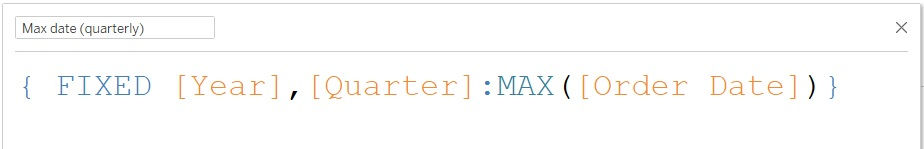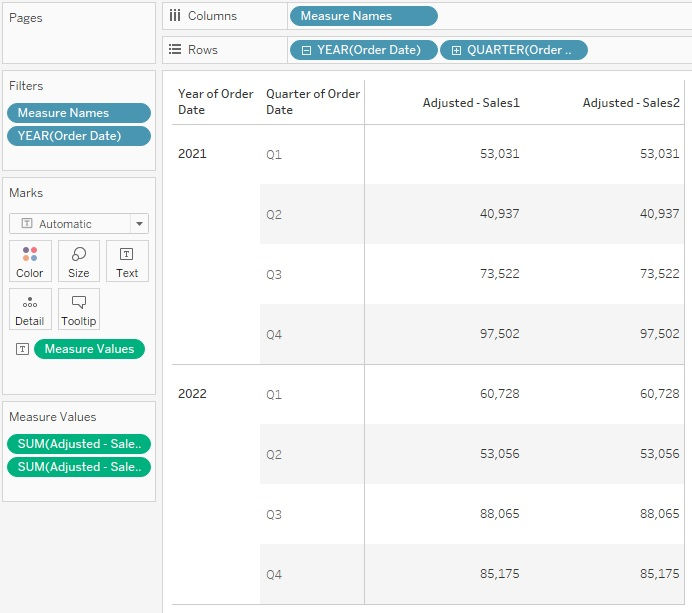top of page
Search
• Bernard K

# Displaying quarterly results as last month’s values for each quarter in Tableau### Introduction

This article demonstrates how to compute quarterly results as last month’s values for each quarter, e.g., Q1 results as March results, Q2 results as June results, Q3 results as September results, and Q4 results as December results (assuming fiscal year start is January).

To demonstrate this, I’ll be using the Sample-Superstore data to compute the quarterly sales metrics as the last month’s values.

To do that, first create a calculated field that returns sales value for months we’re interested in (March, June, September, and December) using any of the two options below.

### Option1

Using the following calculation.(This calculation returns the sales value in the respective months we’re interested in)

### Option2

First compute the maximum date in each quarter using the following calculation.Next compute the sales values whenever the month of maximum date is equal to the date (Order Date) using the following calculation.Notice the above two calculations returns the same results when added to the view below.(Which returns sales values for the last month of each quarter)

Consequently, becoming the quarter sales when presented at quarter level.With the adjusted sales value now, you can compute QTD and PQTD using ideas shared in one of the previous articles on date calculations.

### Conclusion

If this post was helpful and you would like to receive more of these Tableau tips and tricks, kindly subscribe to our mailing list below.

If you like the work we do and would like to work with us, drop us an email on our Contacts page and we’ll reach out!